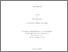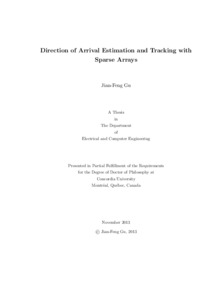Title:

# Direction of Arrival Estimation and Tracking with Sparse Arrays

Gu, Jian Feng (2013) Direction of Arrival Estimation and Tracking with Sparse Arrays. PhD thesis, Concordia University.Preview Text (application/pdf) Gu_PhD_S2014.pdf - Accepted Version Available under License Spectrum Terms of Access. 3MB

## Abstract

Direction of Arrival (DOA) estimation and tracking of a plane wave or multiple plane waves impinging on an array of sensors from noisy data are two of the most important tasks in array signal processing, which have attracted tremendous research interest over the past several decades. It is well-known that the estimation accuracy, angular resolution, tracking capacity, computational complexity, and hardware implementation cost of a DOA estimation and/or tracking technique depend largely on the array geometry. Large arrays with many sensors provide accurate DOA estimation and perfect target tracking, but they usually suffer from a high cost for hardware implementation. Sparse arrays can yield similar DOA estimates and tracking performance with fewer elements for the same-size array aperture as compared to the traditional uniform arrays. In addition, the signals of interest may have rich temporal information that can be exploited to effectively eliminate background noise and significantly improve the performance and capacity of DOA estimation and tracking, and/or even dramatically reduce the computational burden of estimation and tracking algorithms. Therefore, this thesis aims to provide some solutions to improving the DOA estimation and tracking performance by designing sparse arrays and exploiting prior knowledge of the incident signals such as AR modeled sources and known waveforms.

First, we design two sparse linear arrays to efficiently extend the array aperture and improve the DOA estimation performance. One scheme is called minimum redundancy sparse subarrays (MRSSA), where the subarrays are used to obtain an extended correlation matrix according to the principle of minimum redundancy linear array (MRLA). The other linear array is constructed using two sparse ULAs, where the inter-sensor spacing within the same ULA is much larger than half wavelength. Moreover, we propose a 2-D DOA estimation method based on sparse L-shaped arrays, where the signal subspace is selected from the noise-free correlation matrix without requiring the eigen-decomposition to estimate the elevation angle, while the azimuth angles are estimated based on the modified total least squares (TLS) technique.

Second, we develop two DOA estimation and tracking methods for autoregressive (AR) modeled signal source using sparse linear arrays together with Kalman filter and LS-based techniques. The proposed methods consist of two common stages: in the first stage, the sources modeled by AR processes are estimated by the celebrated Kalman filter and in the second stage, the efficient LS or TLS techniques are employed to estimate the DOAs and AR coefficients simultaneously. The AR-modeled sources can provide useful temporal information to handle cases such as the ones, where the number of sources is larger than the number of antennas. In the first method, we exploit the symmetric array to transfer a complex-valued nonlinear problem to a real-valued linear one, which can reduce the computational complexity, while in the second method, we use the ordinary sparse arrays to provide a more accurate DOA estimation.

Finally, we study the problem of estimating and tracking the direction of arrivals (DOAs) of multiple moving targets with known signal source waveforms and unknown gains in the presence of Gaussian noise using a sparse sensor array. The core idea is to consider the output of each sensor as a linear regression model, each of whose coefficients contains a pair of DOAs and gain information corresponding to one target. These coefficients are determined by solving a linear least squares problem and then updating recursively, based on a block QR decomposition recursive least squares (QRD-RLS) technique or a block regularized LS technique. It is shown that the coefficients from different sensors have the same amplitude, but variable phase information for the same signal. Then, simple algebraic manipulations and the well-known generalized least squares (GLS) are used to obtain an asymptotically-optimal DOA estimate without requiring a search over a large region of the parameter space.

Divisions: Concordia University > Gina Cody School of Engineering and Computer Science > Electrical and Computer Engineering Thesis (PhD) Gu, Jian Feng Concordia University Ph. D. Electrical and Computer Engineering 4 December 2013 Zhu, Wei Ping and Swamy, M.N.S 978563 JIANFENG GU 16 Jun 2014 13:42 18 Jan 2018 17:47
All items in Spectrum are protected by copyright, with all rights reserved. The use of items is governed by Spectrum's terms of access.

Repository Staff Only: item control pageResearch related to the current document (at the CORE website)
Back to top